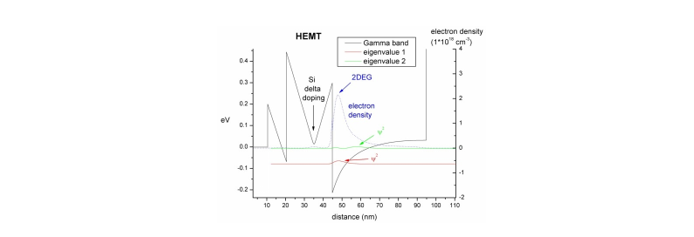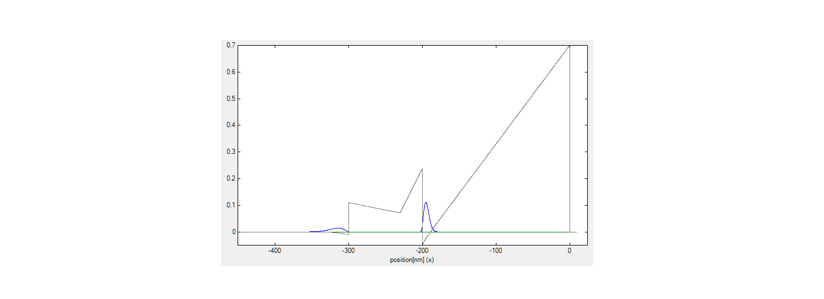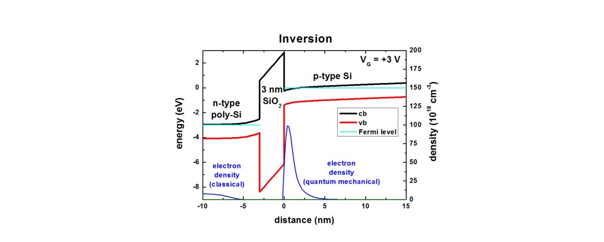# Transistors

## Schrödinger-PoissonWhat you can learn:
• Heterostructure, doping, 2DEG, AlGaAs/GaAs, user-defined function for the doping profile
• Self-consistent Schrödinger-Poisson equation

## HEMTInGaAs/AlInAs HEMT structure (High Electron Mobility Transistor)

What you can learn:
• Heterostructure, doping, 2DEG, InGaAs/AlInAs
• Strain equation, self-consistent Schrödinger-Poisson equation

## Inverted HEMTAlGaAs/GaAs inverted HEMT structure (High Electron Mobility Transistor)

What you can learn:
• heterostructure, doping, 2DEG, AlGaAs/GaAs, variables in input file
• self-consistent Schrödinger-Poisson equation

## AlGaN/GaN FETWhat you can learn:
• Wurtzite, strain, piezoelectricity, pyroelectricity, electron energy levels and wave functions, 2DEG, variables in input file
• Strain equation, self-consistent Schrödinger-Poisson equation

## Ultrathin-body Double Gate FET - DG MOSFET (Double Gate Metal Oxide Semiconductor Field Effect Transistor)DGMOS Structure 1 - Double Gate MOSFET (2D)
(Metal Oxide Semiconductor Field Effect Transistor)
DGMOS Structure 2 - Double Gate MOSFET (2D)
(including quantum mechanics)

What you can learn:
• 2D and 3D simulations, drift-diffusion, mobility models
• self-consistent Current-Poisson equation in 2D and 3D

## Capacitance-Voltage (MIS)What you can learn:
• apply a bias
• self-consistent Schrödinger-Poisson equation

## Doping

Doped semiconductors - Charge carrier concentration as a function of temperature in Si, Ge, GaAs

## 1D MOSFET

p-Si / SiO2 / poly-Si structure (MOSFET with inversion channel due to applied gate voltage)